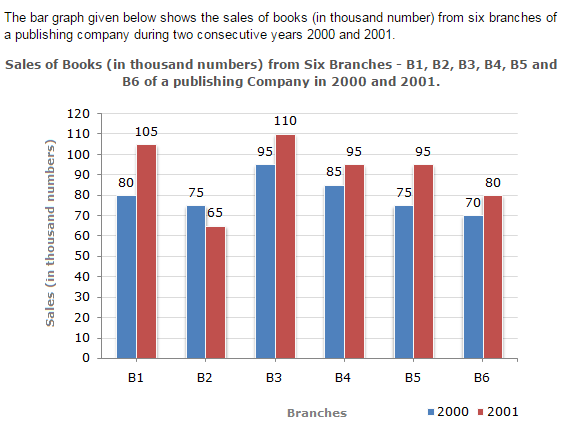Direction1.

What is the ratio of the total sales of branch B2 for both years to the total sales of branch B4 for both years?

 A. 2:3 B. 3:5 C. 4:5 D. 7:9
Explanation: Required ratio = (75 + 65) = 140 = 7 . (85 + 95) 180 9

2.

Total sales of branch B6 for both the years is what percent of the total sales of branches B3 for both the years?

 A. 68.54% B. 71.11% C. 73.17% D. 75.55%
Explanation: Required percentage = [ (70 + 80) x 100 ] % (95 + 110) = [ 150 x 100 ] % 205 = 73.17%.

3.

What percent of the average sales of branches B1, B2 and B3 in 2001 is the average sales of branches B1, B3 and B6 in 2000?

 A. 75% B. 77.5% C. 82.5% D. 87.5%
Explanation: Average sales (in thousand number) of branches B1, B3 and B6 in 2000 = 1 x (80 + 95 + 70) = ( 245 ) . 3 3 Average sales (in thousand number) of branches B1, B2 and B3 in 2001 = 1 x (105 + 65 + 110) = ( 280 ) . 3 3 Therefore Required percentage = [ 245/3 x 100 ] % = ( 245 x 100 ) % = 87.5%. 280/3 280

4.

What is the average sales of all the branches (in thousand numbers) for the year 2000?

 A. 73 B. 80 C. 83 D. 88